Next: Grid OCL : A Graphical Object Connecting Language
Up: Applications
Previous: An IRAF Port of the New IUE Calibration Pipeline

## GIM2D: An IRAF package for the Quantitative Morphology Analysis of Distant Galaxies

Luc Simard
UCO/Lick Observatory, Kerr Hall, University of California, Santa Cruz, CA 95064, Email: simard@ucolick.org

### Abstract:

This paper describes the capabilities of GIM2D, an IRAF package for the quantitative morphology analysis of distant galaxies. GIM2D automatically decomposes all the objects on an input image as the sum of a Sérsic profile and an exponential profile. Each decomposition is then subtracted from the input image, and the results are a galaxy-free'' image and a catalog of quantitative structural parameters. The heart of GIM2D is the Metropolis Algorithm which is used to find the best parameter values and their confidence intervals through Monte-Carlo sampling of the likelihood function. GIM2D has been successfully used on a wide range of datasets: the Hubble Deep Field, distant galaxy clusters and compact narrow-emission line galaxies.

### 1. Introduction

GIM2D (Galaxy IMage 2D) is an IRAF package written to perform detailed bulge/disk decompositions of low signal-to-noise images of distant galaxies.
GIM2D consists of 14 tasks which can be used to fit 2D galaxy profiles, build residual images, simulate realistic galaxies, and determine multidimensional galaxy selection functions. This paper gives an overview of GIM2D capabilities.

### 2. Image Modelling

The first component (bulge'') of the 2D surface brightness used by GIM2D to model galaxy images is a Sérsic (1968) profile of the form: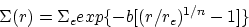(1)
where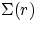is the surface brightness at r. The parameter b is chosen so that re remains the projected radius enclosing half of the light in this component. Thus, the classical de Vaucouleurs profile has n = 4. The second component (disk'') is an exponential profile of the form: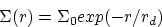(2)
where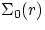is the central surface brightness, and rd is the disk scale length. The model has a total of 12 parameters: the total luminosity L, the bulge fraction B/T, the bulge effective radius re, the bulge ellipticity e, the bulge position angle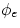, the Sérsic index n, the disk scale length rd, the disk inclination i, the disk position angle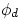, the centroiding offsets dx and dy, and the residual sky background level db.

Detector undersampling (as in the HST/WFPC2 camera) is taken into account by generating the surface brightness model on an oversampled grid, convolving it with the appropriate Point-Spread-Function (PSF) and rebinning the result to the detector resolution.

### 3. Parameter Search with the Metropolis Algorithm

The 12-dimensional parameter space can have a very complicated topology with local minima at low S/N ratios. It is therefore important to choose an algorithm which does not easily get fooled by those local minima. The Metropolis Algorithm (Metropolis et al. 1953) was designed to search for parameter values in a complicated topology. Compared to gradient search methods, the Metropolis is not efficient i.e., it is CPU intensive. On the other hand, gradient searches are greedy. They will start from initial parameter values, dive in the first minimum they encounter and claim it is the global one. The Metropolis does its best to avoid this trap.

The Metropolis in GIM2D starts from an initial set of parameters given by the image moments of the object and computes the likelihood P(w|D,M) that the parameter set w is the true one given the data D and the model M. It then generates random perturbations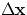about that initial location with a given temperature''. When the search is hot'', large perturbations are tried. After each trial perturbation, the Metropolis computes the likelihood value P1 at the new location, and immediately accepts the trial perturbation if P1 is greater than the old value P0. However, if P1 < P0, then the Metropolis will accept the trial perturbation only P1/P0 of the time. Therefore, the Metropolis will sometime accept trial perturbations which take it to regions of lower likelihood, and this apparently strange behavior is very valuable. If the Metropolis finds a minimum, it will try to get out of it, but it will only have a finite probability (related to the depth of the minimum) of succeeding.

The step matrix for the trial perturbationsis given by the simple equation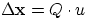where the vector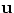consists of randomly generated numbers between 0 and 1, and the matrix Q is obtained through Choleski inversion of the local covariance matrix. In short, the sampling of parameter space shapes itself to the local topology.

Convergence is achieved when the difference between two likelihood values separated by 100 iterations is less than 3-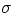of the likelihood fluctuations. After convergence, the Metropolis Monte-Carlo samples the region where the likelihood is thus maximized and stores the accepted parameter sets as it goes along to build the distribution P(w|D,M). Once the region has been sufficiently sampled, the Metropolis computes the median of P(w|D,M) for each model parameter as well as the 99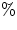confidence limits. The output of the fitting process consists of a PSF-convolved model image O, a residual image R, and a log file containing all Metropolis iterations, the final parameter values and their confidence intervals, and image asymmetry indices.

### 4. Image Reduction Pipeline

GIM2D has a simple image reduction pipeline for HST (or ground-based) images which makes GIM2D particularly suitable for the analysis of archival data:

• Obtain archival data, combine image stacks and remove cosmic rays. Cosmic rays can be removed using tasks such as STSDAS/CRREJ.
• Run SExtractor (Bertin & Arnouts 1996) on the science image to produce an object catalog and a segmentation'' image. Pixel values in this segmentation image indicate to which object a given pixel belongs. The segmentation image is used by GIM2D to deblend galaxy images.
• For HST images, Data Quality Files obtained with a science image stack can be combined and apply to the SExtractor segmentation image. Bad pixels identified by the DQF images are excluded from the bulge/disk decompositions.
• Create a Point-Spread-Function (PSF) for each object. Tiny Tim can be used for HST images. GIM2D accepts three types of PSFs: Gaussian, Tiny Tim and user-specified.
• Run bulge/disk decompositions. These decompositions are run under an IRAF script which can be sent to multiple computers so that they can simultaneously work on the same science image.
• Create a residual image. The GIM2D task GRESIDUAL creates a galaxy-subtracted version of the input image à la DAOPHOT. GRESIDUAL sums up all the output galaxy model images and subtracts this sum from the original image. The resulting residual image is extremely useful to visually inspect the residual images of hundreds of objects at a glance. See Figure 1.
• Determine galaxy selection function. The observed distribution of a parameter (e.g., bulge fraction) derived from a large number of objects in an image is the intrinsic distribution multiplied by the galaxy selection function which gives the probability that an object with a given set of structural parameters will be detected by SExtractor. GIM2D determines the galaxy selection function by taking an empty background section of the science image, adding simulated galaxies to that image section and running SExtractor to determine whether it would have been detected or not. The resulting multidimensional selection function can then be used to flat-field'' the observed structural parameter distributions.

### 5.1. Hubble Deep Field

Hubble Deep Field F814W images were analyzed with GIM2D (Marleau and Simard 1998). The number of bulge-dominated galaxies is much lower (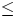10) than indicated by qualitative morphological studies. Some of these studies quoted bulge-dominated galaxy fraction as high as 30(van den Bergh et al. 1996). It was also found that a number of HDF galaxies have surface brightness profiles with Sérsic index n1. Some of them exhibit double exponential profiles which can be easily mistaken for pure bulge systems. However, these systems have spectra resembling those of late-type star-forming galaxies. Other HDF objects are best-fitted with a single n < 1 component.

### 5.2. The Distant Cluster CL0024+16

The rich cluster CL0024+16 at z = 0.4 was the perfect testing ground for the galaxy image deblending in GIM2D. CL0024+16 had been extensively studied for gravitational lensing effects, but a quantitative morphological analysis had never been performed. A total of 560 objects were analyzed in two colors with GIM2D, and about 260 objects were large and/or bright enough to produce reliable bulge/disk decompositions. Figure 1 shows a WFPC2/F814W image of a section of CL0024+16 and the corresponding GIM2D residual image.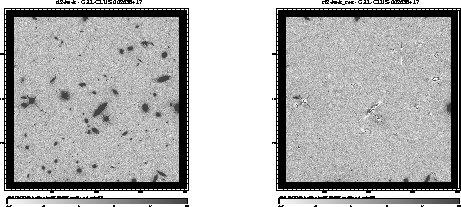### References:

Bertin, E. & Arnouts, S. 1996, A&AS, 117, 393

Marleau, F., & Simard, L. 1998, in preparation

Metropolis N., Rosenbluth, N., Rosenbluth, A., Teller, A., & Teller, E. 1953, Journal of Chemical Physics, 21, 1087

Sérsic, J.-L. 1968, Atlas de Galaxias Australes, Observatorio Astronomico, Cordoba

van den Bergh, S., Abraham, R., Ellis, R.S., Tanvir, N.R., Santiago, B.X., & Glazebrook, K.G. 1996, AJ, 112, 359

© Copyright 1998 Astronomical Society of the Pacific, 390 Ashton Avenue, San Francisco, California 94112, USA

Next: Grid OCL : A Graphical Object Connecting Language
Up: Applications
Previous: An IRAF Port of the New IUE Calibration Pipeline